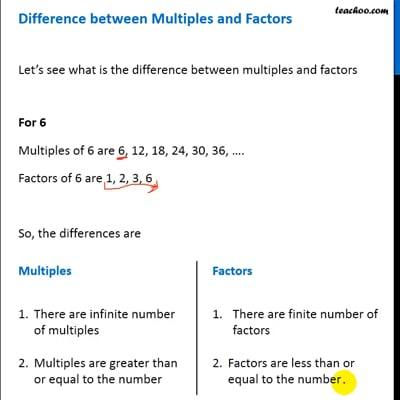Factors and Multiples

Chapter 3 Class 6 Playing with Numbers
Concept wise

Let’s see what is the difference between multiples and factors

For 6

Multiples of 6 are 6, 12, 18, 24, 30, 36, ….

Factors of 6 are 1, 2, 3, 6

So, the differences are

 Multiples Factors 1.There are infinite number of multiples 2.Multiples are greater than or equal to the number 1.There are finite number of factors 2.Factors are less than or equal to the numberThis video is only available for Teachoo black users

### Transcript

Multiples There are infinite number of multiples Multiples are greater than or equal to the number Factors There are finite number of factors Factors are less than or equal to the number